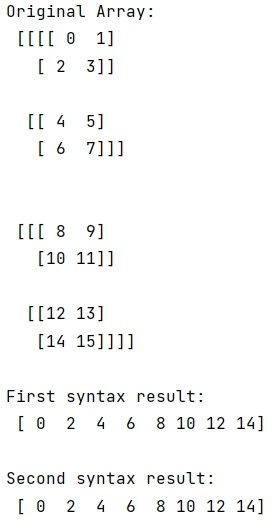# How do you use the ellipsis slicing syntax?

Learn, how to use the ellipsis slicing syntax in Python?
Submitted by Pranit Sharma, on December 26, 2022

NumPy is an abbreviated form of Numerical Python. It is used for different types of scientific operations in python. Numpy is a vast library in python which is used for almost every kind of scientific or mathematical operation. It is itself an array which is a collection of various methods and functions for processing the arrays.

## Ellipsis slicing

The ellipsis is used in NumPy to slice high-dimensional data structures. It is designed to mean at this point, inserting as many full slices (:) to extend the multi-dimensional slice to all dimensions.

Suppose that you are given a four-dimensional matrix of order 2 X 2 X 2 x 2. To select all the first elements in the fourth dimension we can use the ellipsis notation.

Ellipses are also useful for zero-dimensional data structures. They are the only way I know of to write into scalar NumPy ndarrays. The sentence of ellipsis slicing is not a hidden feature of python, it is just a constant. The only point is at no built-in method or Python language constructor makes use of this.

But, according to its documentation, NumPy uses it. There is another use for ellipsis, which has nothing to do with slices. It can be used in intra-thread communication with queues, as a mark that signals "Done".

Let us understand with the help of an example,

## Python code to demonstrate the use the ellipsis slicing syntax

```# Import numpy
import numpy as np

# Creating a numpy array
arr = np.arange(16).reshape(2,2,2,2)

# Display original array
print("Original Array:\n",arr,"\n")

# Using first ellipses syntax
res = arr[..., 0].flatten()

# Display result
print("First syntax result:\n",res,"\n")

# Using second ellipses syntax
res = arr[:,:,:,0].flatten()

# Display result
print("Second syntax result:\n",res,"\n")
```

Output: